# Proof Review CS 202 Aaron Bloomfield Spring 2007

• Slides: 53
Download presentationProof Review CS 202 Aaron Bloomfield Spring 2007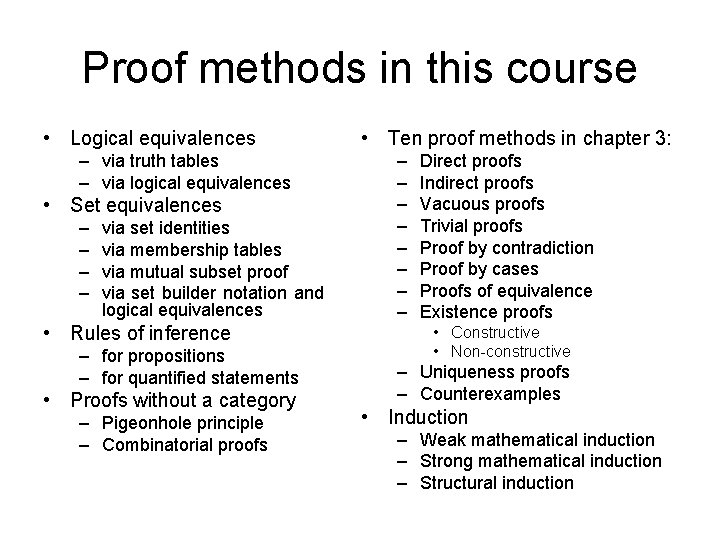Proof methods in this course • Logical equivalences – via truth tables – via logical equivalences • Set equivalences – – via set identities via membership tables via mutual subset proof via set builder notation and logical equivalences • Rules of inference – for propositions – for quantified statements • Proofs without a category – Pigeonhole principle – Combinatorial proofs • Ten proof methods in chapter 3: – – – – Direct proofs Indirect proofs Vacuous proofs Trivial proofs Proof by contradiction Proof by cases Proofs of equivalence Existence proofs • Constructive • Non-constructive – Uniqueness proofs – Counterexamples • Induction – Weak mathematical induction – Strong mathematical induction – Structural inductionLogic proofsUsing Truth Tables p q r p→r q →r (p→r) (q →r) p q (p q) →r T T T T T F F T F T T F F F T T T F T F T F F T T F T F F F T T T F T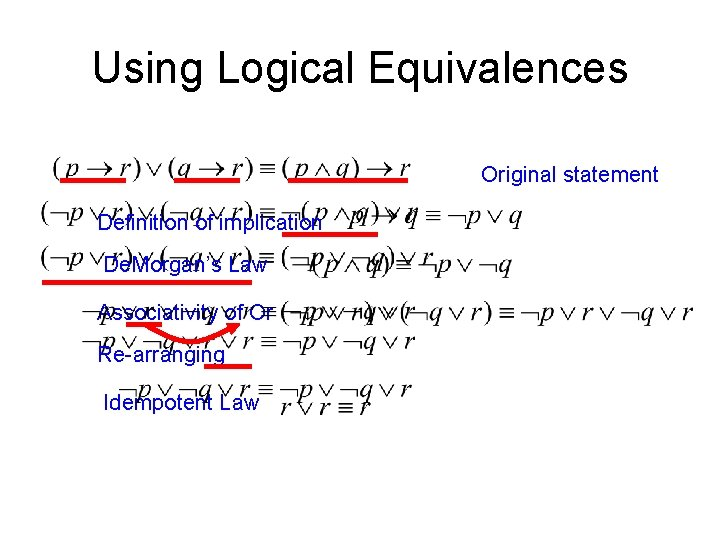Using Logical Equivalences Original statement Definition of implication De. Morgan’s Law Associativity of Or Re-arranging Idempotent LawSet proofsProof by using basic set identities • Prove that A∩B=B-(B-A) Definition of difference De. Morgan’s law Complementation law Distributive law Complement law Identity law Commutative law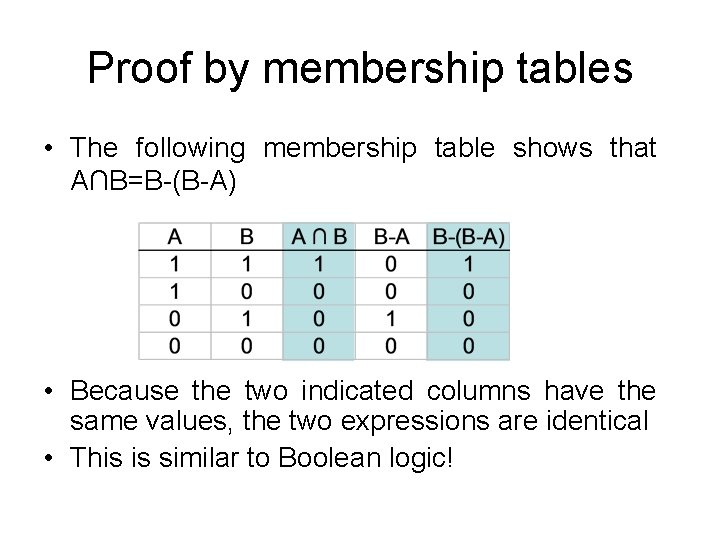Proof by membership tables • The following membership table shows that A∩B=B-(B-A) • Because the two indicated columns have the same values, the two expressions are identical • This is similar to Boolean logic!Proof by showing each set is a subset of the other 1 • Assume that x B-(B-A) – By definition of difference, we know that x B and x B-A • Consider x B-A – If x B-A, then (by definition of difference) x B and x A – Since x B-A, then only one of the inverses has to be true (De. Morgan’s law): x B or x A • So we have that x B and (x B or x A) – It cannot be the case where x B and x B – Thus, x B and x A – This is the definition of intersection • Thus, if x B-(B-A) then x A∩B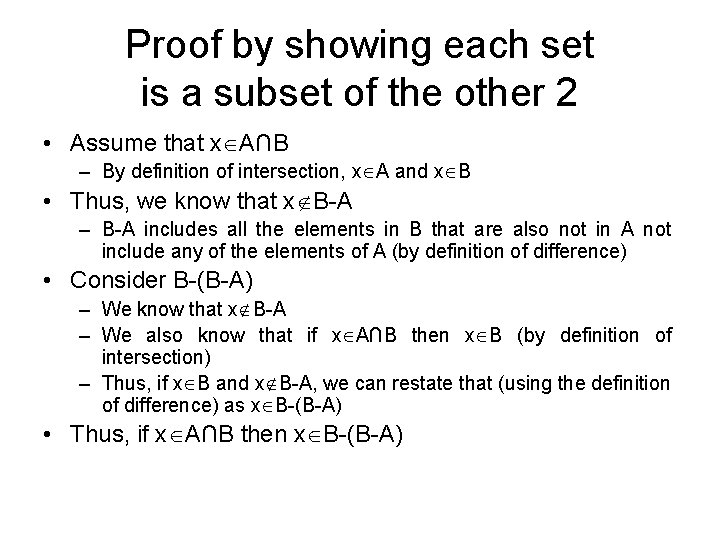Proof by showing each set is a subset of the other 2 • Assume that x A∩B – By definition of intersection, x A and x B • Thus, we know that x B-A – B-A includes all the elements in B that are also not in A not include any of the elements of A (by definition of difference) • Consider B-(B-A) – We know that x B-A – We also know that if x A∩B then x B (by definition of intersection) – Thus, if x B and x B-A, we can restate that (using the definition of difference) as x B-(B-A) • Thus, if x A∩B then x B-(B-A)Proof by set builder notation and logical equivalences 2 Original statement Definition of difference Negating “element of” Definition of difference De. Morgan’s Law Distributive Law Negating “element of” Negation Law Identity Law Definition of intersectionRules of InferenceModus Ponens example • Assume you are given the following two statements: – “you are in this class” – “if you are in this class, you will get a grade” • Let p = “you are in this class” • Let q = “you will get a grade” • By Modus Ponens, you can conclude that you will get a grade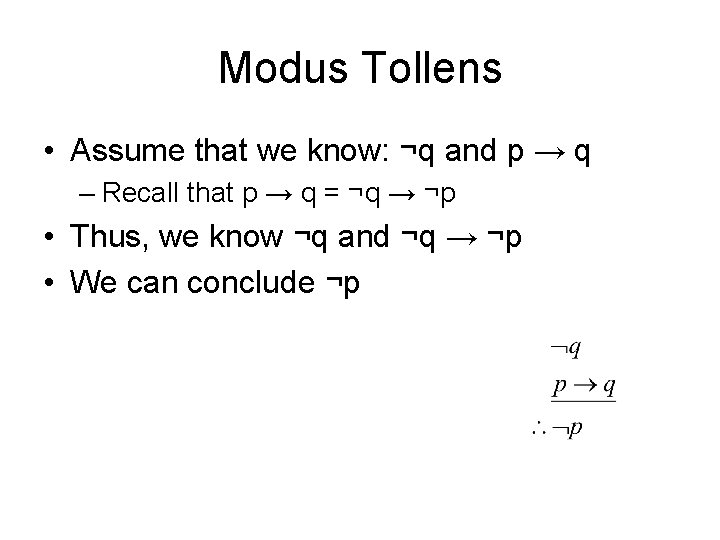Modus Tollens • Assume that we know: ¬q and p → q – Recall that p → q = ¬q → ¬p • Thus, we know ¬q and ¬q → ¬p • We can conclude ¬p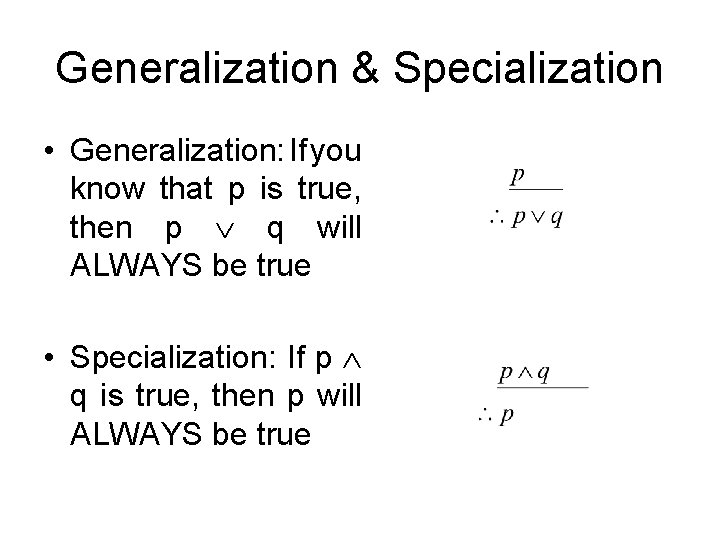Generalization & Specialization • Generalization: If you know that p is true, then p q will ALWAYS be true • Specialization: If p q is true, then p will ALWAYS be trueMore rules of inference • Conjunction: if p and q are true separately, then p q is true • Elimination: If p q is true, and p is false, then q must be true • Transitivity: If p→q is true, and q→r is true, then p→r must be true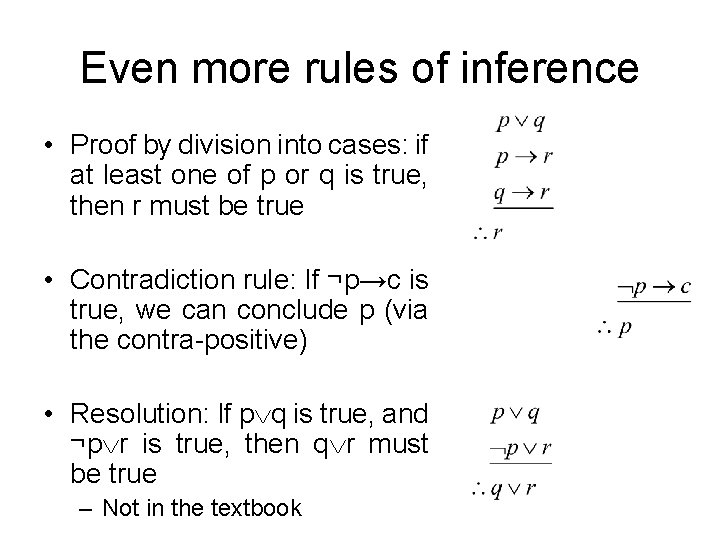Even more rules of inference • Proof by division into cases: if at least one of p or q is true, then r must be true • Contradiction rule: If ¬p→c is true, we can conclude p (via the contra-positive) • Resolution: If p q is true, and ¬p r is true, then q r must be true – Not in the textbookExample of proof • • We have the hypotheses: p q r s t – “It is not sunny this afternoon and it is colder than yesterday” – “We will go swimming only if it is sunny” – “If we do not go swimming, then we will take a canoe trip” – “If we take a canoe trip, then we will be home by sunset” • Does this imply that “we will be home by sunset”? ¬p q r→p ¬r → s s→t tExample of proof 1. 2. 3. 4. 5. 6. 7. 8. ¬p q ¬p r→p ¬r ¬r → s s s→t t 1 st hypothesis Simplification using step 1 2 nd hypothesis Modus tollens using steps 2 & 3 3 rd hypothesis Modus ponens using steps 4 & 5 4 th hypothesis Modus ponens using steps 6 & 7Example of proof • Given the hypotheses: – “If it does not rain or if it is not foggy, then the sailing race will be held and the lifesaving demonstration will go on” – “If the sailing race is held, then the trophy will be awarded” – “The trophy was not awarded” • Can you conclude: “It rained”? (¬r ¬f) → (s l) s→t ¬t r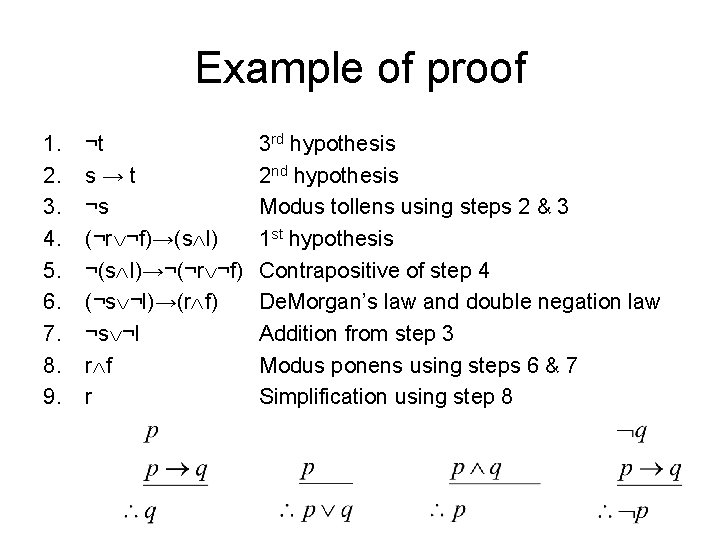Example of proof 1. 2. 3. 4. 5. 6. 7. 8. 9. ¬t s→t ¬s (¬r ¬f)→(s l) ¬(s l)→¬(¬r ¬f) (¬s ¬l)→(r f) ¬s ¬l r f r 3 rd hypothesis 2 nd hypothesis Modus tollens using steps 2 & 3 1 st hypothesis Contrapositive of step 4 De. Morgan’s law and double negation law Addition from step 3 Modus ponens using steps 6 & 7 Simplification using step 8Proofs without a categoryCorollary 1: algebraic proof Let n be a non-negative integer. Then Algebraic proof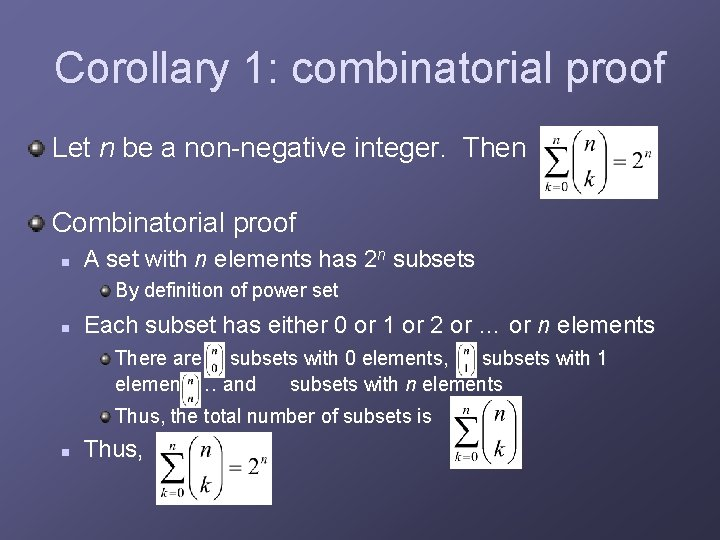Corollary 1: combinatorial proof Let n be a non-negative integer. Then Combinatorial proof n A set with n elements has 2 n subsets By definition of power set n Each subset has either 0 or 1 or 2 or … or n elements There are subsets with 0 elements, subsets with 1 element, … and subsets with n elements Thus, the total number of subsets is n Thus,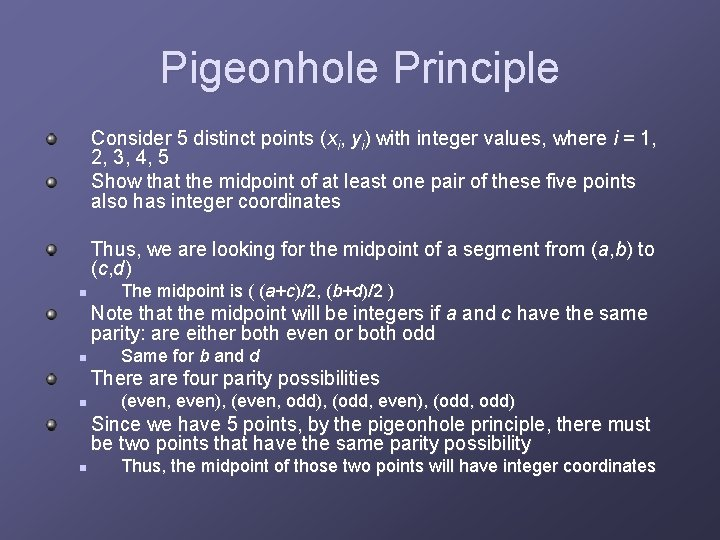Pigeonhole Principle Consider 5 distinct points (xi, yi) with integer values, where i = 1, 2, 3, 4, 5 Show that the midpoint of at least one pair of these five points also has integer coordinates Thus, we are looking for the midpoint of a segment from (a, b) to (c, d) n The midpoint is ( (a+c)/2, (b+d)/2 ) Note that the midpoint will be integers if a and c have the same parity: are either both even or both odd n Same for b and d There are four parity possibilities n (even, even), (even, odd), (odd, even), (odd, odd) Since we have 5 points, by the pigeonhole principle, there must be two points that have the same parity possibility n Thus, the midpoint of those two points will have integer coordinatesTen proof methods in chapter 3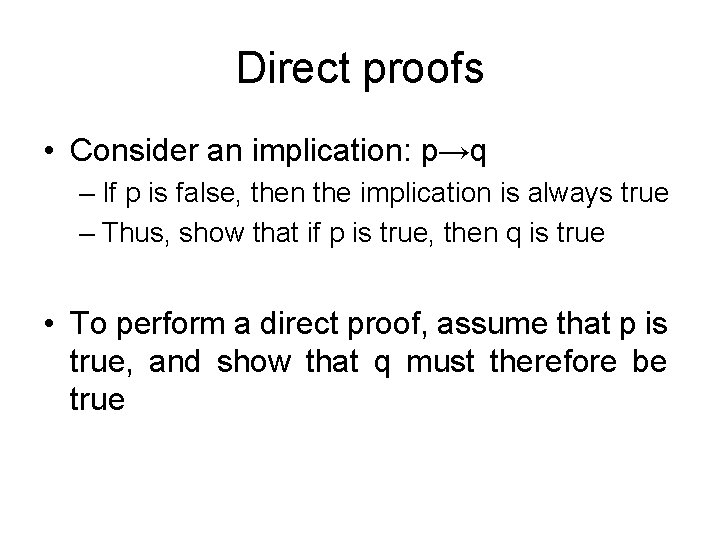Direct proofs • Consider an implication: p→q – If p is false, then the implication is always true – Thus, show that if p is true, then q is true • To perform a direct proof, assume that p is true, and show that q must therefore be trueDirect proof example • Show that the square of an even number is an even number – Rephrased: if n is even, then n 2 is even • Assume n is even – Thus, n = 2 k, for some k (definition of even numbers) – n 2 = (2 k)2 = 4 k 2 = 2(2 k 2) – As n 2 is 2 times an integer, n 2 is thus even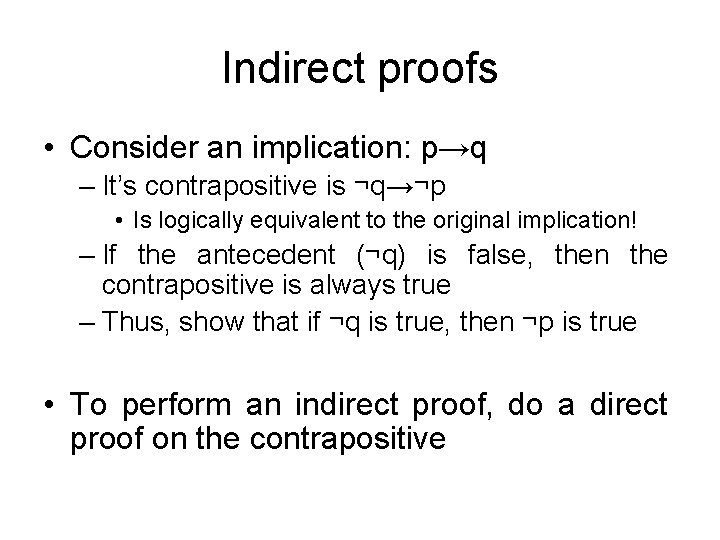Indirect proofs • Consider an implication: p→q – It’s contrapositive is ¬q→¬p • Is logically equivalent to the original implication! – If the antecedent (¬q) is false, then the contrapositive is always true – Thus, show that if ¬q is true, then ¬p is true • To perform an indirect proof, do a direct proof on the contrapositiveIndirect proof example • If n 2 is an odd integer then n is an odd integer • Prove the contrapositive: If n is an even integer, then n 2 is an even integer • Proof: n=2 k for some integer k (definition of even numbers) • n 2 = (2 k)2 = 4 k 2 = 2(2 k 2) • Since n 2 is 2 times an integer, it is even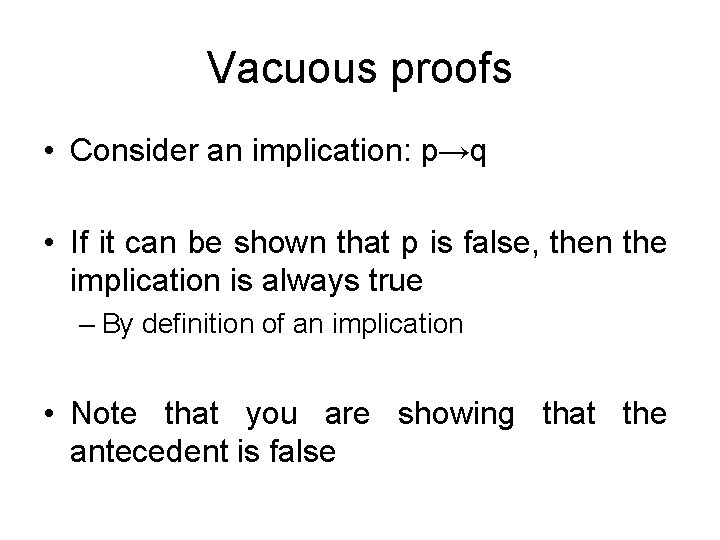Vacuous proofs • Consider an implication: p→q • If it can be shown that p is false, then the implication is always true – By definition of an implication • Note that you are showing that the antecedent is falseVacuous proof example • Consider the statement: – All criminology majors in CS 202 are female – Rephrased: If you are a criminology major and you are in CS 202, then you are female • Could also use quantifiers! • Since there are no criminology majors in this class, the antecedent is false, and the implication is trueTrivial proofs • Consider an implication: p→q • If it can be shown that q is true, then the implication is always true – By definition of an implication • Note that you are showing that the conclusion is true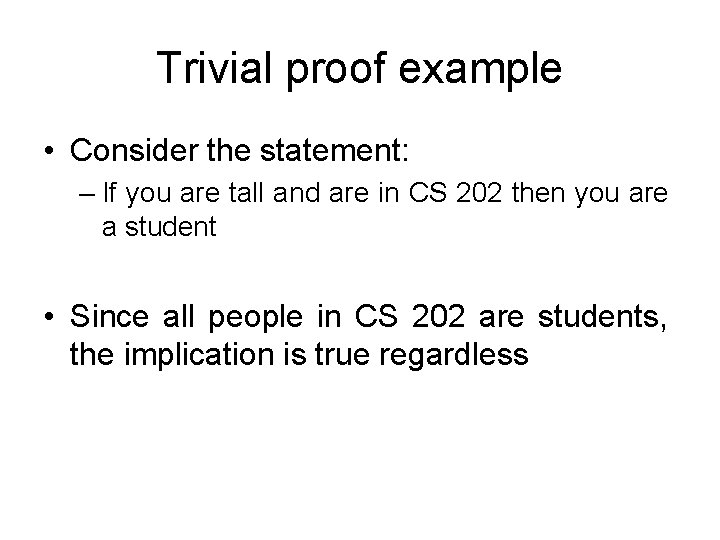Trivial proof example • Consider the statement: – If you are tall and are in CS 202 then you are a student • Since all people in CS 202 are students, the implication is true regardlessProof by contradiction • Given a statement p, assume it is false – Assume ¬p • Prove that ¬p cannot occur – A contradiction exists • Given a statement of the form p→q – To assume it’s false, you only have to consider the case where p is true and q is falseProof by contradiction example • Theorem (by Euclid): There are infinitely many prime numbers. • Proof. Assume there a finite number of primes • List them as follows: p 1, p 2 …, pn. • Consider the number q = p 1 p 2 … pn + 1 – This number is not divisible by any of the listed primes • If we divided pi into q, there would result a remainder of 1 – We must conclude that q is a prime number, not among the primes listed above • This contradicts our assumption that all primes are in the list p 1, p 2 …, pn.Proof by cases • Show a statement is true by showing all possible cases are true • Thus, you are showing a statement of the form: is true by showing that: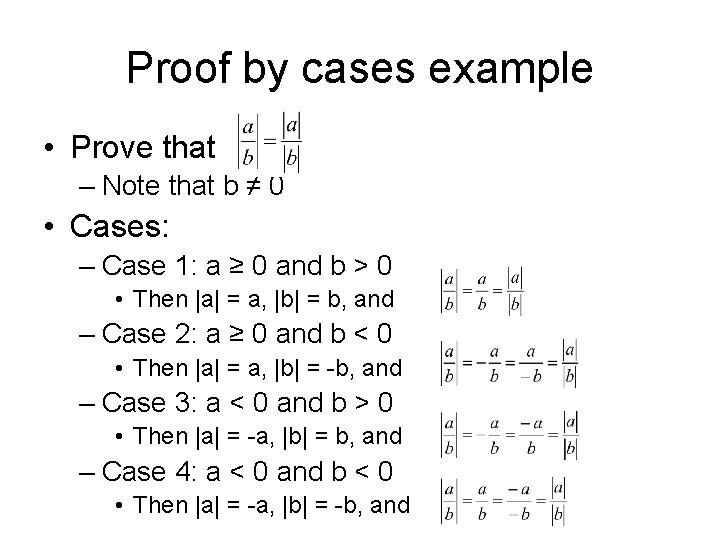Proof by cases example • Prove that – Note that b ≠ 0 • Cases: – Case 1: a ≥ 0 and b > 0 • Then |a| = a, |b| = b, and – Case 2: a ≥ 0 and b < 0 • Then |a| = a, |b| = -b, and – Case 3: a < 0 and b > 0 • Then |a| = -a, |b| = b, and – Case 4: a < 0 and b < 0 • Then |a| = -a, |b| = -b, and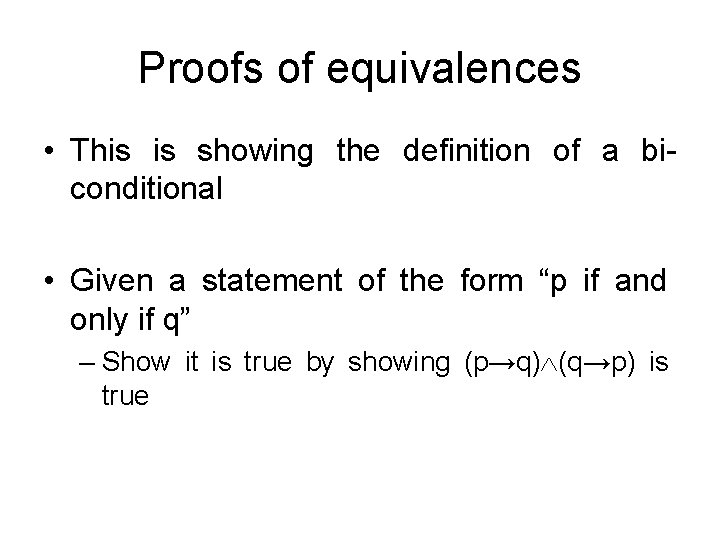Proofs of equivalences • This is showing the definition of a biconditional • Given a statement of the form “p if and only if q” – Show it is true by showing (p→q) (q→p) is trueProofs of equivalence example • Show that m 2=n 2 if and only if m=n or m=-n – Rephrased: (m 2=n 2) ↔ [(m=n) (m=-n)] • Need to prove two parts: – [(m=n) (m=-n)] → (m 2=n 2) • Proof by cases! • Case 1: (m=n) → (m 2=n 2) – (m)2 = m 2, and (n)2 = n 2, so this case is proven • Case 2: (m=-n) → (m 2=n 2) – (m)2 = m 2, and (-n)2 = n 2, so this case is proven – (m 2=n 2) → [(m=n) (m=-n)] • • Subtract n 2 from both sides to get m 2 -n 2=0 Factor to get (m+n)(m-n) = 0 Since that equals zero, one of the factors must be zero Thus, either m+n=0 (which means m=n) or m-n=0 (which means m=-n)Existence proofs • Given a statement: x P(x) • We only have to show that a P(c) exists for some value of c • Two types: – Constructive: Find a specific value of c for which P(c) exists – Nonconstructive: Show that such a c exists, but don’t actually find it • Assume it does not exist, and show a contradictionConstructive existence proof example • Show that a square exists that is the sum of two other squares – Proof: 32 + 42 = 52 • Show that a cube exists that is the sum of three other cubes – Proof: 33 + 43 + 53 = 63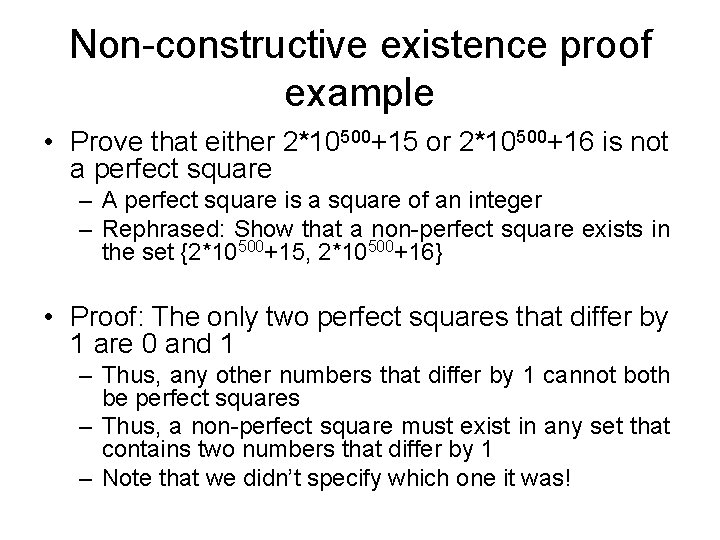Non-constructive existence proof example • Prove that either 2*10500+15 or 2*10500+16 is not a perfect square – A perfect square is a square of an integer – Rephrased: Show that a non-perfect square exists in the set {2*10500+15, 2*10500+16} • Proof: The only two perfect squares that differ by 1 are 0 and 1 – Thus, any other numbers that differ by 1 cannot both be perfect squares – Thus, a non-perfect square must exist in any set that contains two numbers that differ by 1 – Note that we didn’t specify which one it was!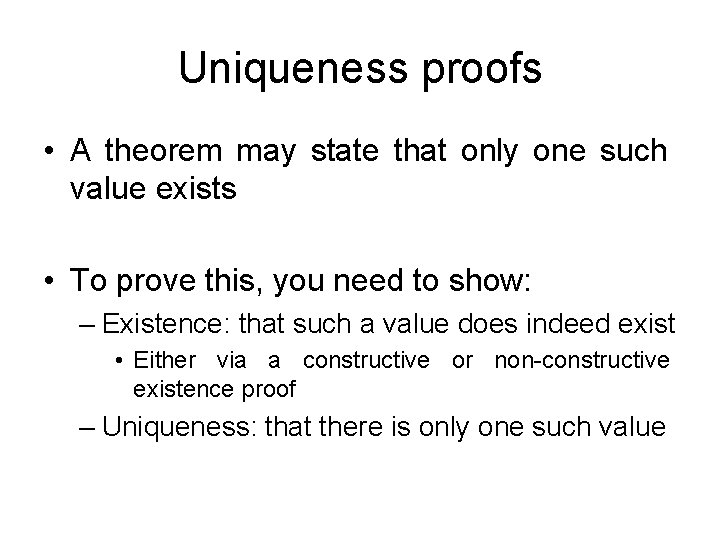Uniqueness proofs • A theorem may state that only one such value exists • To prove this, you need to show: – Existence: that such a value does indeed exist • Either via a constructive or non-constructive existence proof – Uniqueness: that there is only one such value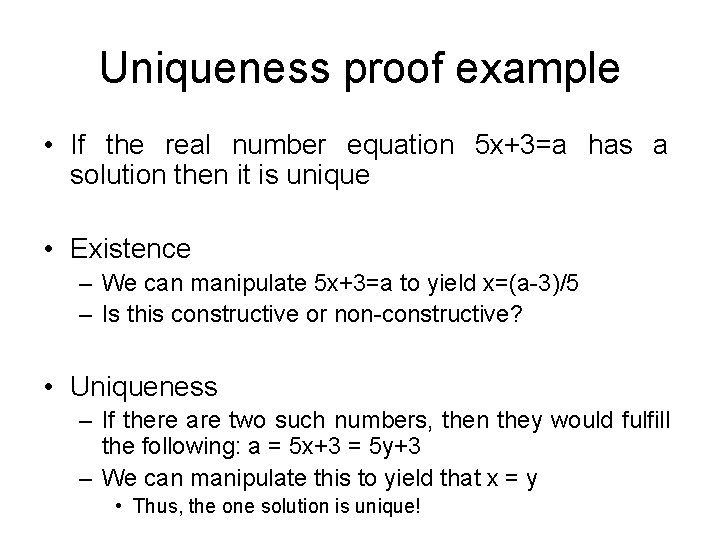Uniqueness proof example • If the real number equation 5 x+3=a has a solution then it is unique • Existence – We can manipulate 5 x+3=a to yield x=(a-3)/5 – Is this constructive or non-constructive? • Uniqueness – If there are two such numbers, then they would fulfill the following: a = 5 x+3 = 5 y+3 – We can manipulate this to yield that x = y • Thus, the one solution is unique!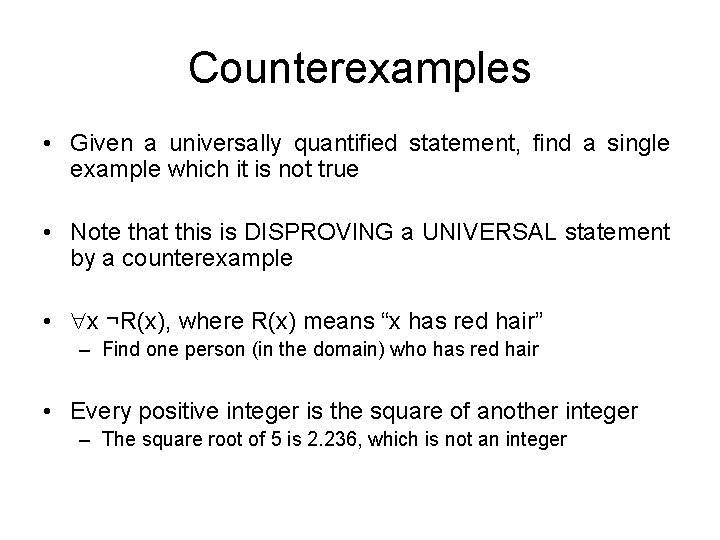Counterexamples • Given a universally quantified statement, find a single example which it is not true • Note that this is DISPROVING a UNIVERSAL statement by a counterexample • x ¬R(x), where R(x) means “x has red hair” – Find one person (in the domain) who has red hair • Every positive integer is the square of another integer – The square root of 5 is 2. 236, which is not an integer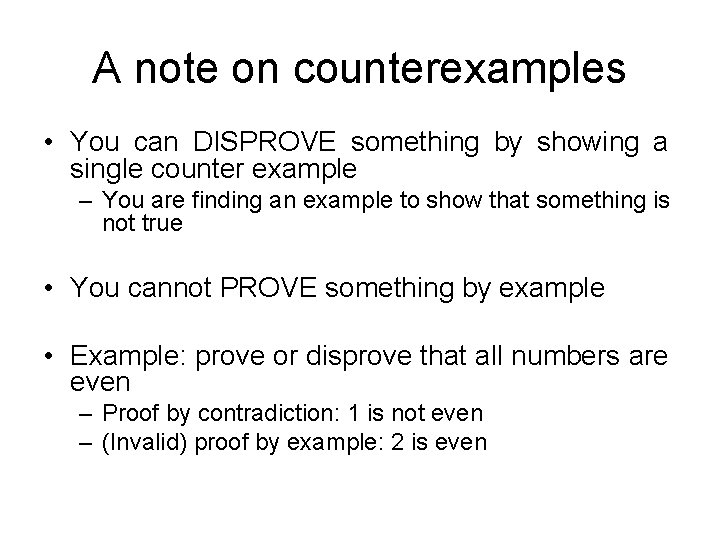A note on counterexamples • You can DISPROVE something by showing a single counter example – You are finding an example to show that something is not true • You cannot PROVE something by example • Example: prove or disprove that all numbers are even – Proof by contradiction: 1 is not even – (Invalid) proof by example: 2 is evenInduction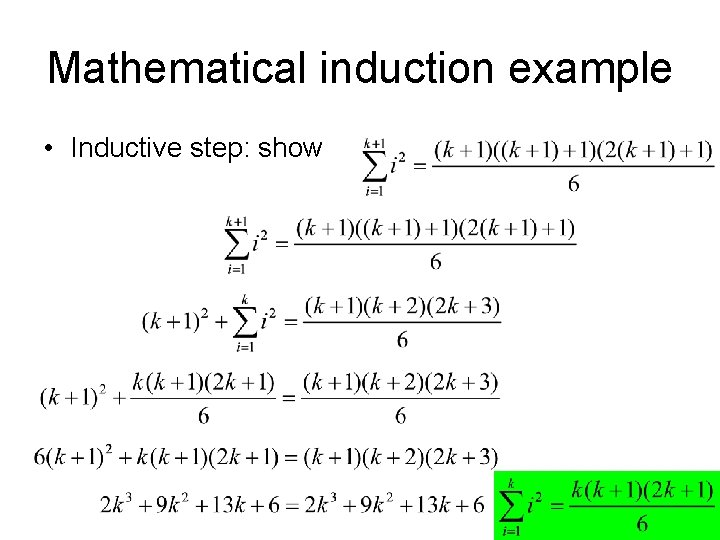Mathematical induction example • Inductive step: showStrong induction example • Show that any number > 1 can be written as the product of one or more primes • Base case: P(2) – 2 is the product of 2 (remember that 1 is not prime!) • Inductive hypothesis: assume P(2), P(3), …, P(k) are all true • Inductive step: Show that P(k+1) is true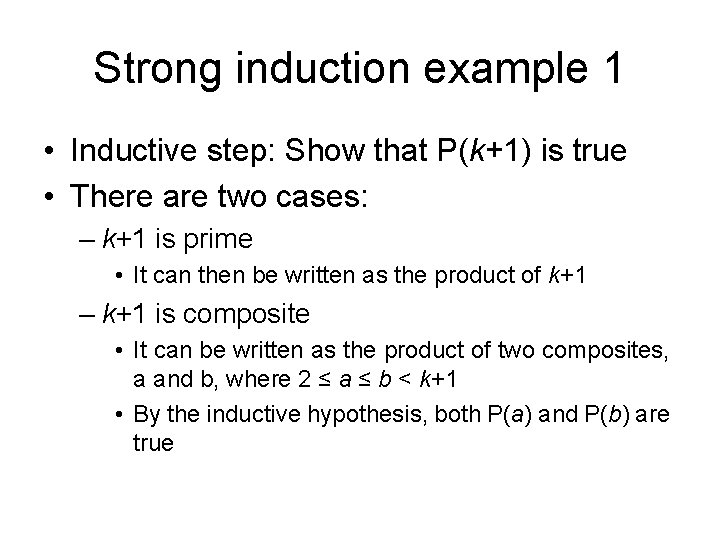Strong induction example 1 • Inductive step: Show that P(k+1) is true • There are two cases: – k+1 is prime • It can then be written as the product of k+1 – k+1 is composite • It can be written as the product of two composites, a and b, where 2 ≤ a ≤ b < k+1 • By the inductive hypothesis, both P(a) and P(b) are true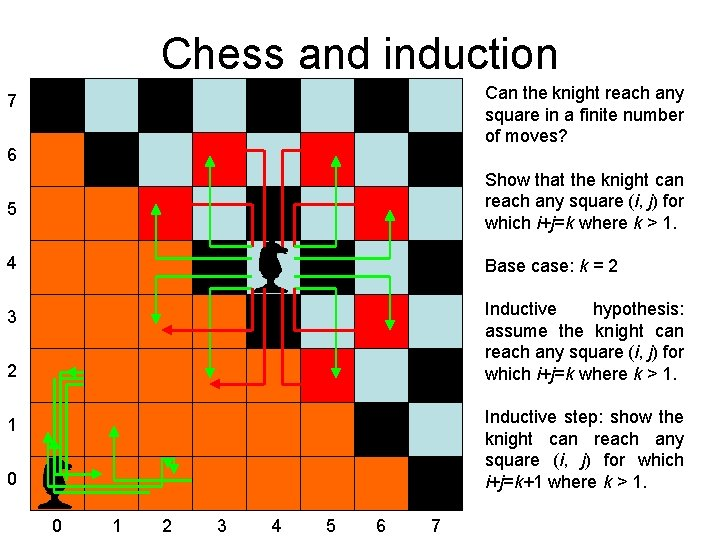Chess and induction Can the knight reach any square in a finite number of moves? 7 6 5 Show that the knight can reach any square (i, j) for which i+j=k where k > 1. 4 Base case: k = 2 3 Inductive hypothesis: assume the knight can reach any square (i, j) for which i+j=k where k > 1. 2 Inductive step: show the knight can reach any square (i, j) for which i+j=k+1 where k > 1. 1 0 0 1 2 3 4 5 6 7Chess and induction • Inductive step: show the knight can reach any square (i, j) for which i+j=k+1 where k > 1. – Note that k+1 ≥ 3, and one of i or j is ≥ 2 – If i ≥ 2, the knight could have moved from (i-2, j+1) • Since i+j = k+1, i-2 + j+1 = k, which is assumed true – If j ≥ 2, the knight could have moved from (i+1, j-2) • Since i+j = k+1, i+1 + j-2 = k, which is assumed true